#14-一级指针和多级指针

0
0
01. 云栖社区>
2. 博客>
3. 正文

## 指针基本概念

• 什么是地址

• 生活中的地址: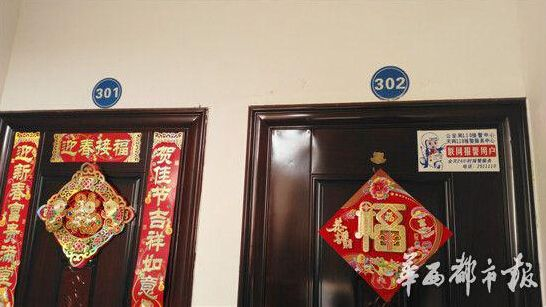• 内存地址: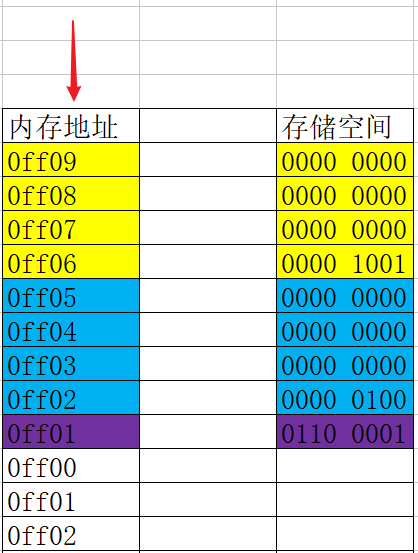• 地址与内存单元中的数据是两个完全不同的概念

• 地址如同房间编号, 根据这个编号我们可以找到对应的房间
• 内存单元如同房间, 房间是专门用于存储数据的
• 变量地址:

• 系统分配给"变量"的"内存单元"的起始地址
``````int num = 6; // 占用4个字节
//那么变量num的地址为: 0ff06

char c = 'a'; // 占用1个字节
//那么变量c的地址为:0ff05
``````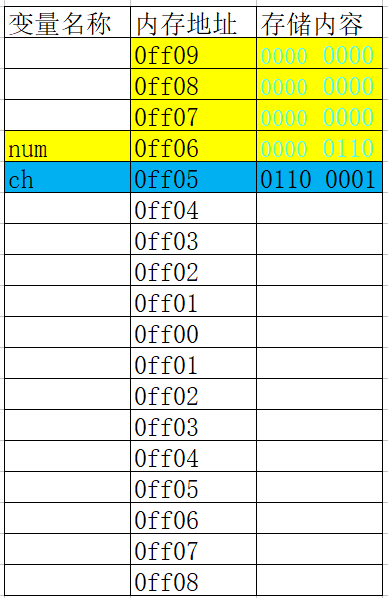## 什么是指针

• 在计算机中所有数据都存储在内存单元中,而每个内存单元都有一个对应的地址, 只要通过这个地址就能找到对应单元中存储的数据.

• 由于通过地址能找到所需的变量单元，所以我们说该地址指向了该变量单元。将地址形象化的称为“指针”

• 内存单元的指针(地址)和内存单元的内容是两个不同的概念。## 什么是指针变量

• 在C语言中,允许用一个变量来存放其它变量的地址, 这种专门用于存储其它变量地址的变量, 我们称之为指针变量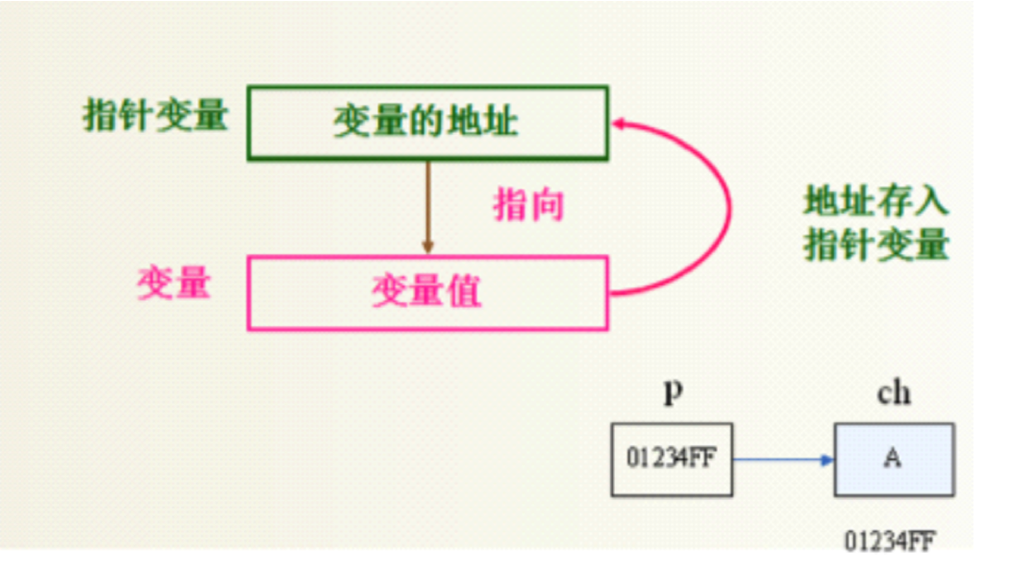• 示例:

``````    int age;// 定义一个普通变量
num = 10;
int *pnAge; // 定义一个指针变量
pnAge = &age;
``````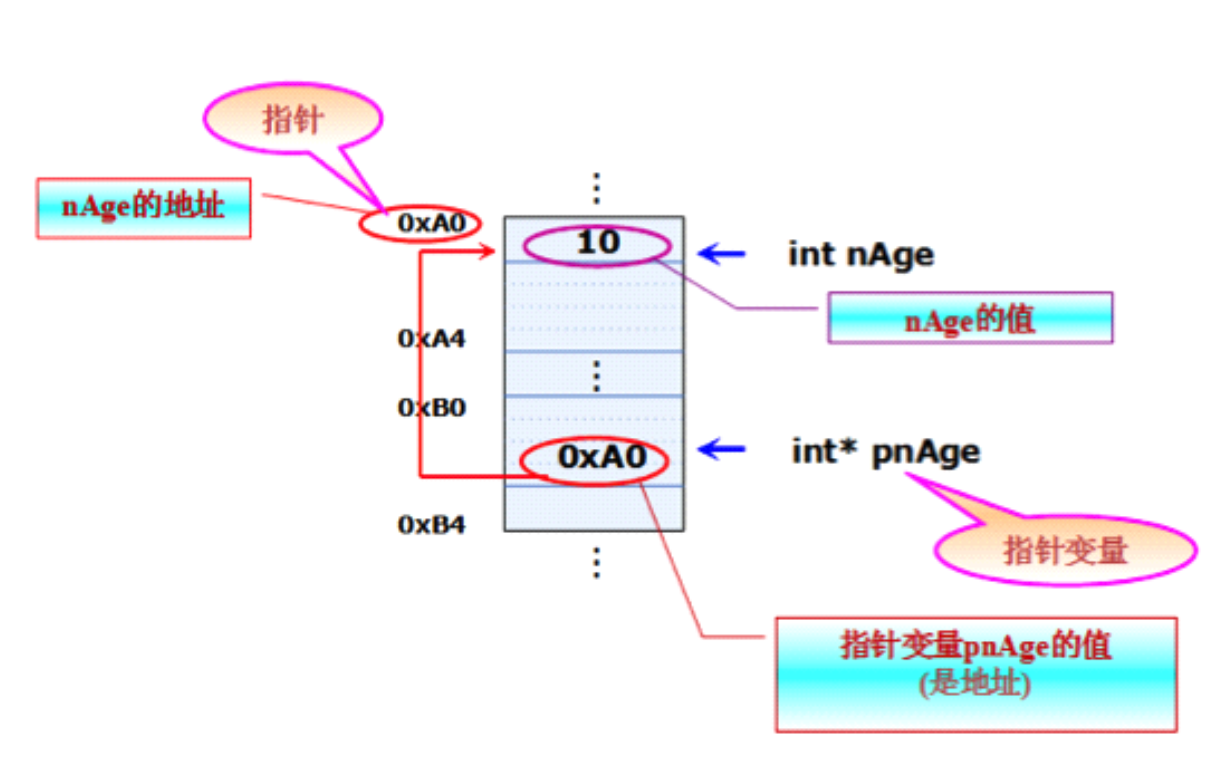## 定义指针变量的格式

• 指针变量的定义包括两个内容:
• 指针类型说明,即定义变量为一个指针变量;
• 指针变量名;• 示例:
``````char ch = 'a';
char *p; // 一个用于指向字符型变量的指针
p = &ch;
int num = 666;
int *q; // 一个用于指向整型变量的指针
q = &num;
``````
• 其中,*表示这是一个指针变量
• 变量名即为定义的指针变量名
• 类型说明符表示本指针变量所指向的变量的数据类型

## 指针变量的初始化方法

• 指针变量初始化的方法有两种:定义的同时进行初始化和先定义后初始化
• 定义的同时进行初始化
``````int a = 5;
int *p = &a;
``````
• 先定义后初始化
``````int a = 5;
int *p;
p=&a;
``````
• 把指针初始化为NULL
``````int *p=NULL;
int *q=0;
``````
• 不合法的初始化:
• 指针变量只能存储地址, 不能存储其它类型
``````int *p;
p =  250; // 错误写法
``````
• 给指针变量赋值时,指针变量前不能再加“*”
``````int *p;
*p=&a; //错误写法
``````
• 注意点:

• 多个指针变量可以指向同一个地址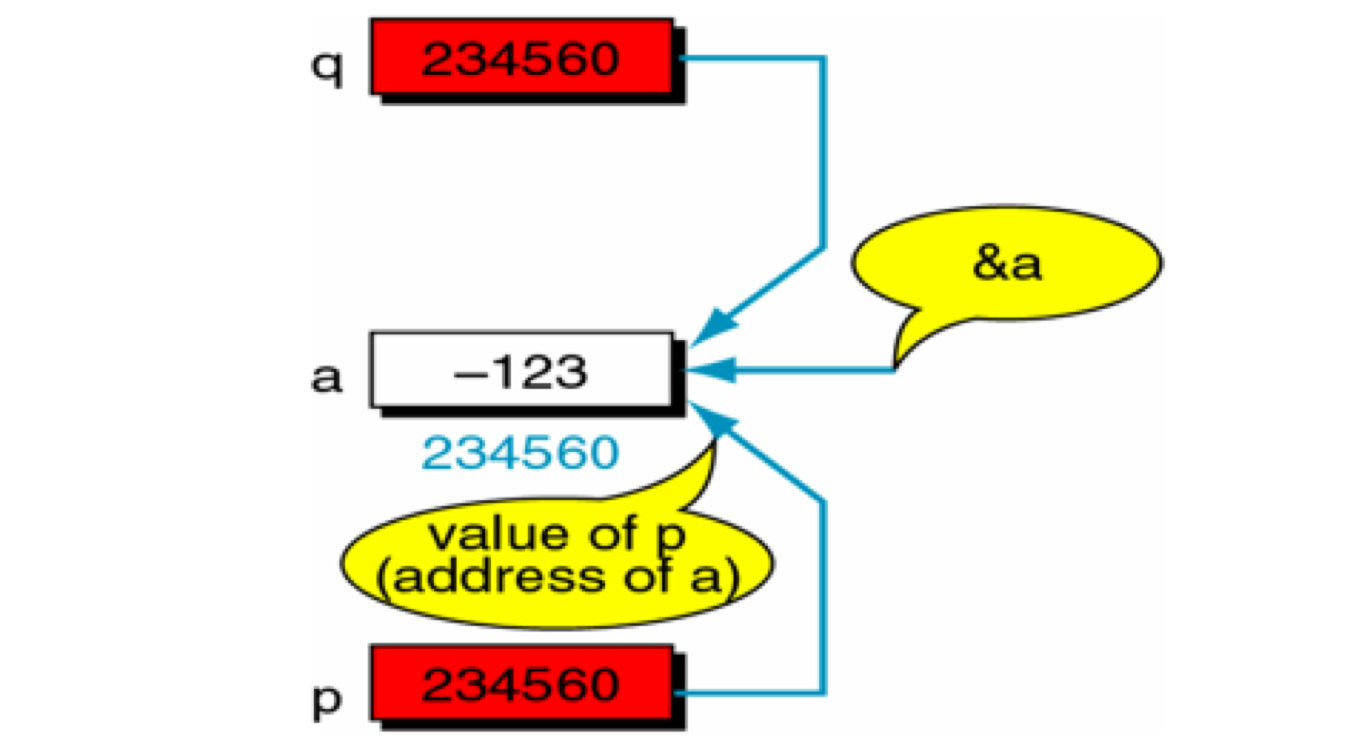• 指针的指向是可以改变的

``````int a = 5;
int *p = &a;
int b = 10;
p = &b; // 修改指针指向
``````
• 指针没有初始化里面是一个垃圾值,这时候我们这是一个野指针
• 野指针可能会导致程序崩溃
• 野指针访问你不该访问数据
• 所以指针必须初始化才可以访问其所指向存储区域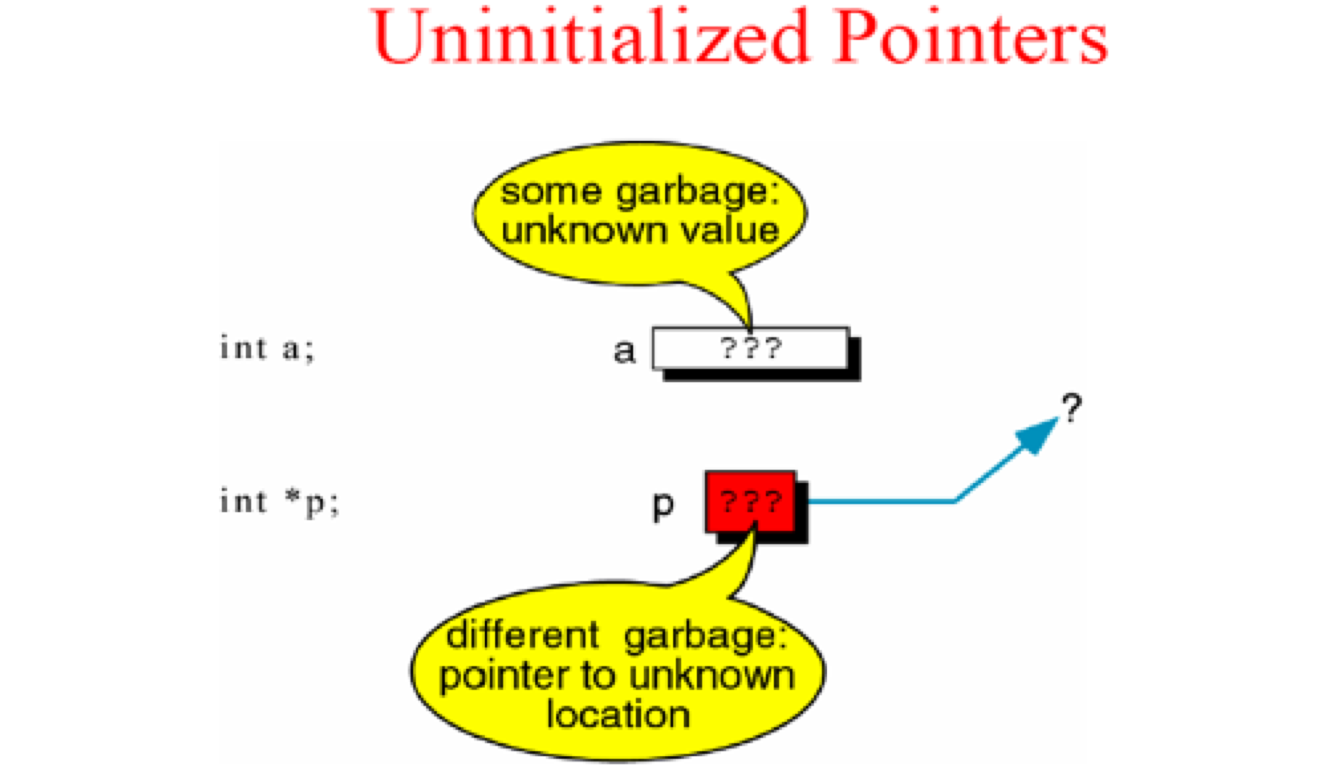## 访问指针所指向的存储空间

• C语言中提供了地址运算符&来表示变量的地址。其一般形式为:
• &变量名;
• C语言中提供了*来定义指针变量和访问指针变量指向的内存存储空间
• 在定义变量的时候 * 是一个类型说明符,说明定义的这个变量是一个指针变量
``````int *p=NULL; // 定义指针变量
``````
• 在不是定义变量的时候 *是一个操作符,代表访问指针所指向存储空间
``````int a = 5;
int *p = &a;
printf("a = %d", *p); // 访问指针变量
``````

## 指针类型

• 在同一种编译器环境下,一个指针变量所占用的内存空间是固定的。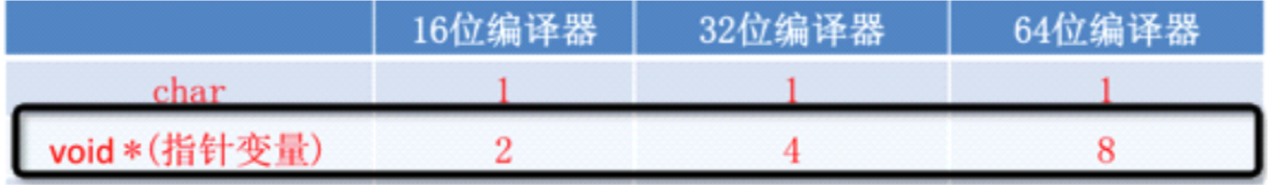• 虽然在同一种编译器下, 所有指针占用的内存空间是一样的,但不同类型的变量却占不同的字节数

• 一个int占用4个字节，一个char占用1个字节，而一个double占用8字节；
• 现在只有一个地址，我怎么才能知道要从这个地址开始向后访问多少个字节的存储空间呢，是4个，是1个，还是8个。
• 所以指针变量需要它所指向的数据类型告诉它要访问多少个字节存储空间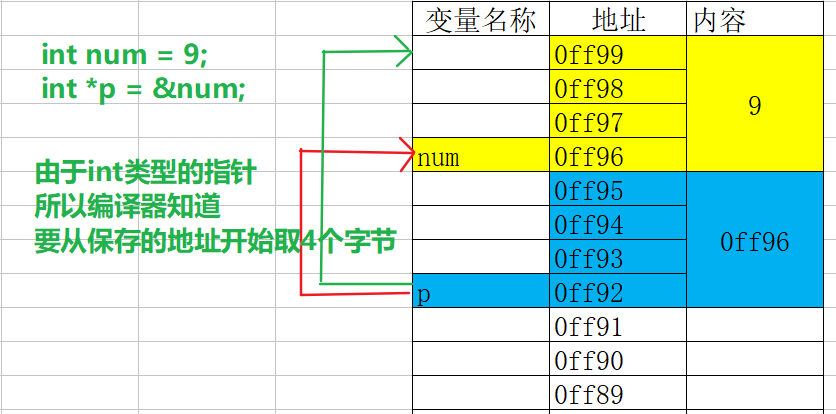## 二级指针

• 如果一个指针变量存放的又是另一个指针变量的地址,则称这个指针变量为指向指针的指针变量。也称为“二级指针”
``````    char c = 'a';
char *cp;
cp = &c;
char **cp2;
cp2 = &cp;
printf("c = %c", **cp2);
``````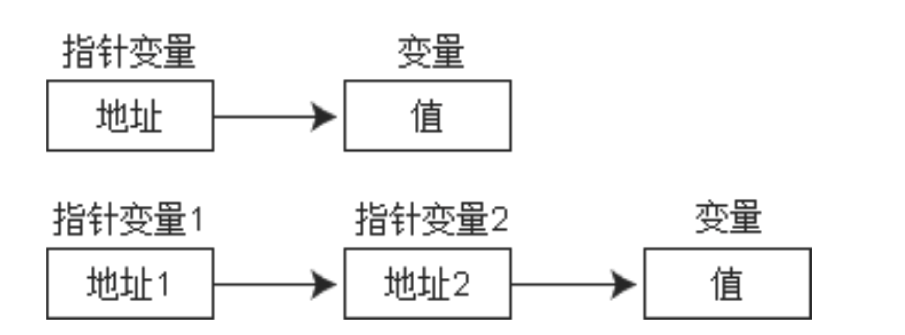• 多级指针的取值规则
``````int ***m1;  //取值***m1
int *****m2; //取值*****m2
``````

## 练习

• 定义一个函数交换两个变量的值
• 写一个函数，同时返回两个数的和与差

## 数组指针的概念及定义

• 数组元素指针
• 一个变量有地址,一个数组包含若干元素,每个数组元素也有相应的地址, 指针变量也可以保存数组元素的地址
• 只要一个指针变量保存了数组元素的地址, 我们就称之为数组元素指针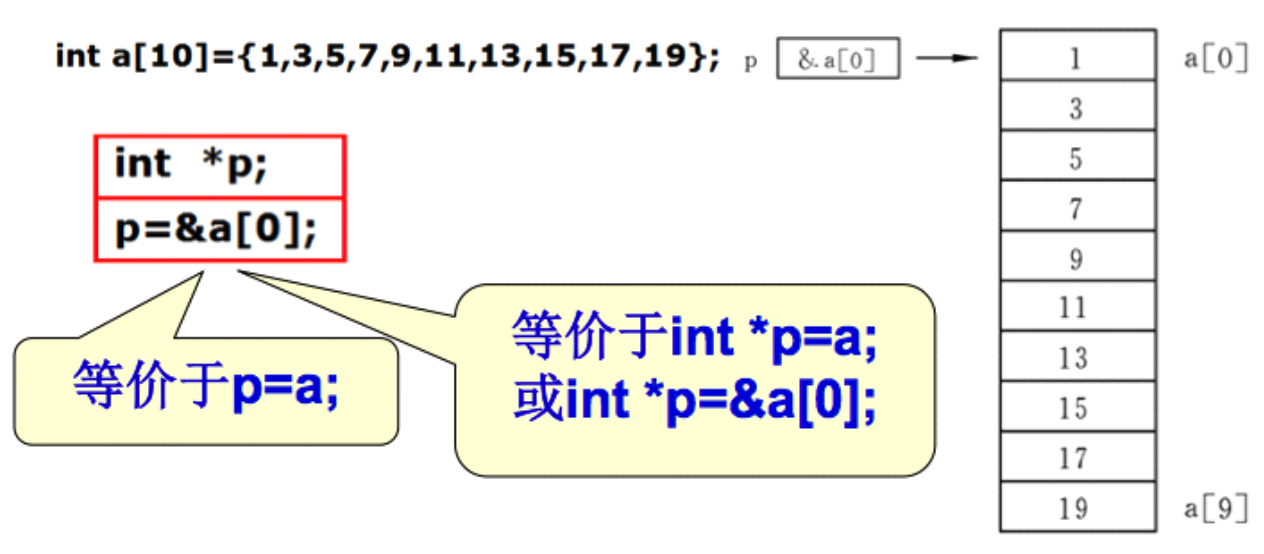``````    printf(“%p %p”, &(a), a); //输出结果:0x1100, 0x1100
``````
• 注意: 数组名a不代表整个数组,只代表数组首元素的地址。
• “p=a;”的作用是“把a数组的首元素的地址赋给指针变量p”,而不是“把数组a各元素的值赋给 p”

## 指针访问数组元素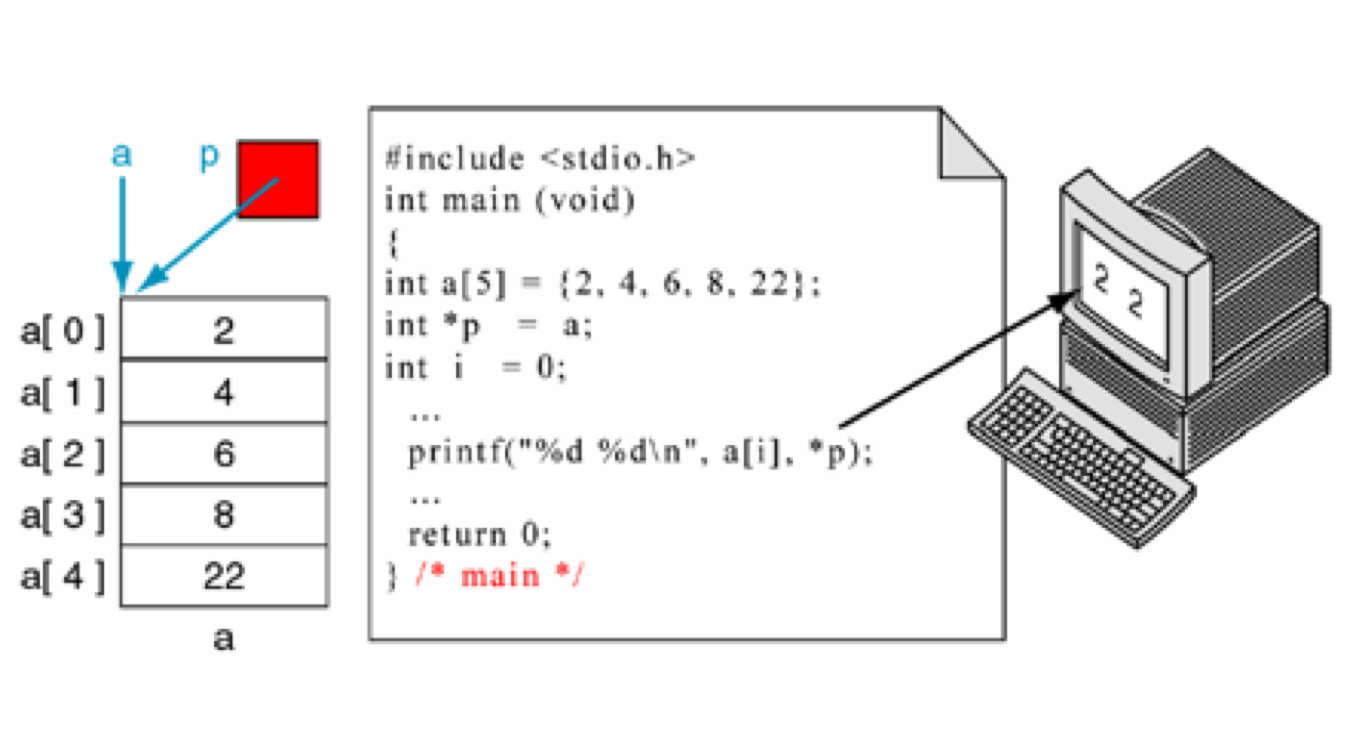``````    int main (void)
{
int a = {2, 4, 6, 8, 22};
int *p;
// p = &(a);
p = a;
printf(“%d %d\n”,a,*p); // 输出结果: 2, 2
}

``````
• 在指针指向数组元素时,允许以下运算:
• 加一个整数(用+或+=),如p+1
• 减一个整数(用-或-=),如p-1
• 自加运算,如p++,++p
• 自减运算,如p--,--p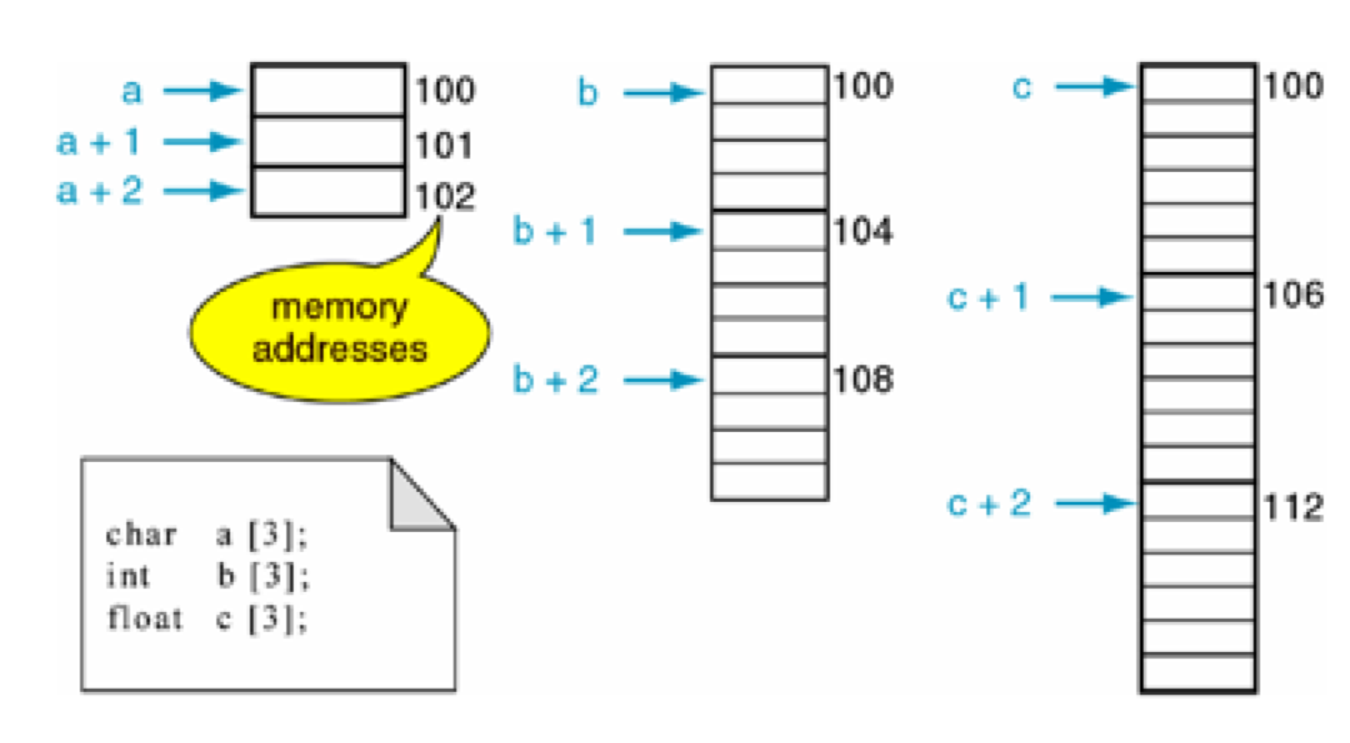• 如果指针变量p已指向数组中的一个元素,则p+1`指向`同一数组中的下一个元素,p-1`指向`同 一数组中的上一个元素。
• 结论: 访问数组元素,可用下面两种方法:
• 下标法, 如a[i]形式
• 指针法, *(p+i)形式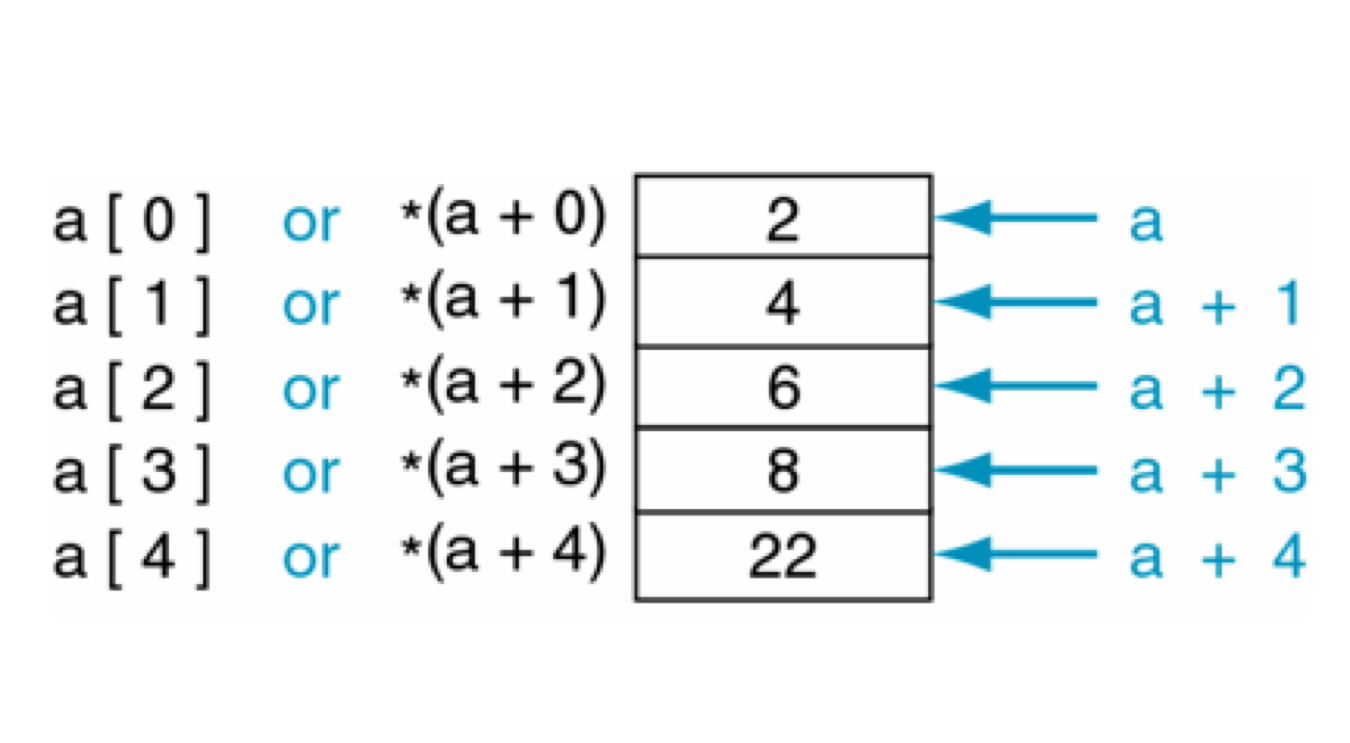• 注意:
• 数组名虽然是数组的首地址，但是数组名所所保存的数组的首地址是不可以更改的
``````    int x;
x++;  //错误
int* p = x;
p++; //正确
``````

## 指针与字符串

• 定义字符串的两种方式
• 字符数组
``````char string[]=”I love lnj!”;
printf("%s\n",string);
``````
• 字符串指针指向字符串
``````// 数组名保存的是数组第0个元素的地址, 指针也可以保存第0个元素的地址
char *str = "abc"
``````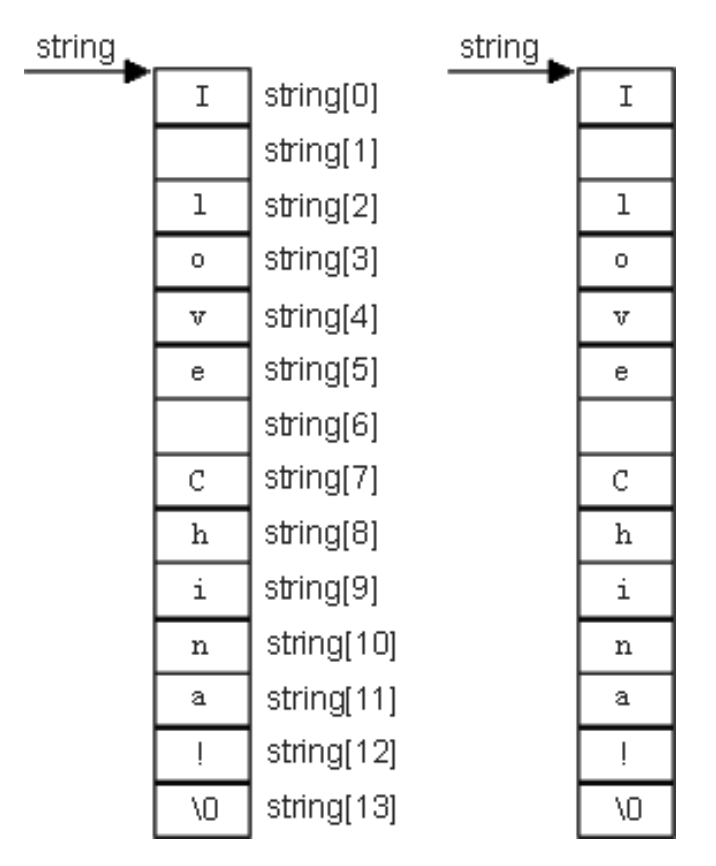• 字符串指针使用注意事项
• 可以查看字符串的每一个字符
``````har *str = "lnj";
for(int i = 0; i < strlen(str);i++)
{
printf("%c-", *(str+i)); // 输出结果:l-n-j
}
``````
• 不可以修改字符串内容
``````//   + 使用字符数组来保存的字符串是保存栈里的,保存栈里面东西是可读可写,所有可以修改字符串中的的字符
//   + 使用字符指针来保存字符串,它保存的是字符串常量地址,常量区是只读的,所以我们不可以修改字符串中的字符
char *str = "lnj";
*(str+2) = 'y'; // 错误
``````
• 不能够直接接收键盘输入
``````// 错误的原因是:str是一个野指针,他并没有指向某一块内存空间
// 所以不允许这样写如果给str分配内存空间是可以这样用 的
char *str;
scanf("%s", str);
``````

## 指向函数指针

• 为什么指针可以指向一个函数？
• 函数作为一段程序，在内存中也要占据部分存储空间，它也有一个起始地址
• 函数有自己的地址，那就好办了，我们的指针变量就是用来存储地址的。
• 因此可以利用一个指针指向一个函数。其中，函数名就代表着函数的地址。
• 指针函数的定义
• 格式: `返回值类型 (*指针变量名)(形参1, 形参2, ...);`
``````    int sum(int a,int b)
{
return a + b;
}

int (*p)(int,int);
p = sum;
``````
• 指针函数定义技巧

• 1、把要指向函数头拷贝过来
• 2、把函数名称使用小括号括起来
• 3、在函数名称前面加上一个*
• 4、修改函数名称
• 应用场景

• 调用函数
• 将函数作为参数在函数间传递
• 注意点:

• 由于这类指针变量存储的是一个函数的入口地址，所以对它们作加减运算(比如p++)是无意义的
• 函数调用中"(指针变量名)"的两边的括号不可少,其中的不应该理解为求值运算,在此处它 只是一种表示符号

+ 关注